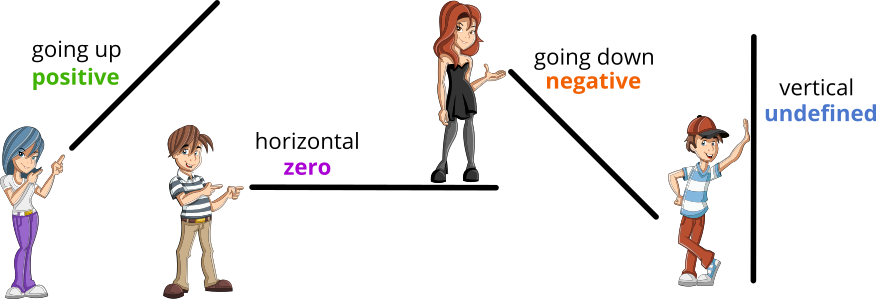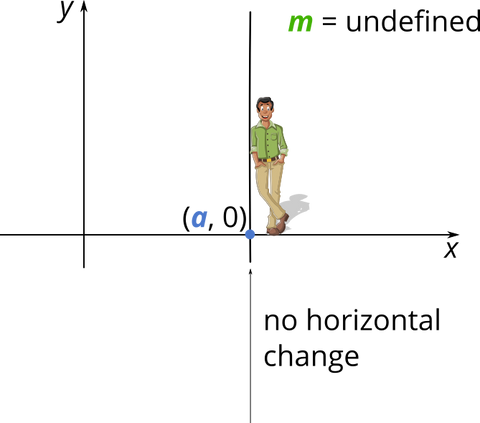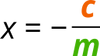## Linear functions - Features

Authored By Carmen Popescu-Rose 0 Comment(s)

Linear functions are functions of the form f(x) = mxc and their graphical representation is a straight line.

m is the slope or the gradient of the line; it represents the steepness of the line.

c is the y - intercept; where the line crosses the y - axisIf the straight line slopes upwards when moving from left to right, then the line has a positive slope or gradient. Equation of line: y = mx + c, where m > 0 Domain: R (the real numbers set) Range: R (the real numbers set)If the straight line slopes downwards when moving from left to right, then the line has a negative slope or gradient. Equation of line: y = mx + c, where m < 0 Domain: R (the real numbers set) Range: R (the real numbers set)If the straight line is horizontal, then the line has a zero slope or gradient, m. Equation of line: y = c, with m = 0 Domain: R (the real numbers set) Range: {c}If the straight line is vertical, then the line has an undefined slope or gradient, m. Equation of line: x = a, with m → ∞   Domain: {a} Range: R (the real numbers set) x-intercept occurs when y = 0 0 = mx + cy-intercept occurs when x = 0 y = m × 0 + c y = c

Tags